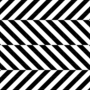Recommended PostsGoogle has beefed up its built-in calculator function, adding an interface with 34 buttons. When a user types a sum such as "2 + 2" or "4 x 5," they are now confronted by the visual interface shown above ? previously, Google would simply spit out the answer as text. The calculator includes functions for processing sines, cosines, and tangents as well as dedicated buttons for Pi and Euler's number.

According to an official Google Twitter account, the company began rolling out the latest stage, 3.9, of its Panda algorithm update last night, but it is not clear whether the addition of the calculator is connected. The calculator is one of a number of features implemented in recent months which seem designed to shift Google's role away from that of a simple search engine and towards a new position as a general tool for answers.

Source: The Verge

Screenshot:Share on other sitesohh...you are right. like it!

Share on other sitesniiiiice

Share on other sitesNow if it only did the graphs too.... lol

Share on other sitesNow if it only did the graphs too.... lol

It does, type in the equation of any graph.

Share on other sitesIt does, type in the equation of any graph.

Yep:Share on other siteshumm..can't see the calculator but can see the graph tool in google.com.

Share on other sitesnice! Good improvement.

Share on other sitesyep graphs work fine

example

cos(12x)

Share on other sites2*sqrt(-abs(abs(x)-1)*abs(3-abs(x))/((abs(x)-1)*(3-abs(x))))(1+abs(abs(x)-3)/(abs(x)-3))sqrt(1-(x/7)^2)+(5+0.97(abs(x-.5)+abs(x+.5))-3(abs(x-.75)+abs(x+.75)))(1+abs(1-abs(x))/(1-abs(x))),-3sqrt(1-(x/7)^2)sqrt(abs(abs(x)-4)/(abs(x)-4)),abs(x/2)-0.0913722(x^2)-3+sqrt(1-(abs(abs(x)-2)-1)^2),(2.71052+(1.5-.5abs(x))-1.35526sqrt(4-(abs(x)-1)^2))sqrt(abs(abs(x)-1)/(abs(x)-1))+0.9
[/CODE]

Cool, though. I use google for math... maybe too often.

Share on other siteshumm..can't see the calculator but can see the graph tool in google.com.

Same here.

Share on other sites2*sqrt(-abs(abs(x)-1)*abs(3-abs(x))/((abs(x)-1)*(3-abs(x))))(1+abs(abs(x)-3)/(abs(x)-3))sqrt(1-(x/7)^2)+(5+0.97(abs(x-.5)+abs(x+.5))-3(abs(x-.75)+abs(x+.75)))(1+abs(1-abs(x))/(1-abs(x))),-3sqrt(1-(x/7)^2)sqrt(abs(abs(x)-4)/(abs(x)-4)),abs(x/2)-0.0913722(x^2)-3+sqrt(1-(abs(abs(x)-2)-1)^2),(2.71052+(1.5-.5abs(x))-1.35526sqrt(4-(abs(x)-1)^2))sqrt(abs(abs(x)-1)/(abs(x)-1))+0.9
[/CODE]

Cool, though. I use google for math... maybe too often.

Too slick!

Share on other sites2*sqrt(-abs(abs(x)-1)*abs(3-abs(x))/((abs(x)-1)*(3-abs(x))))(1+abs(abs(x)-3)/(abs(x)-3))sqrt(1-(x/7)^2)+(5+0.97(abs(x-.5)+abs(x+.5))-3(abs(x-.75)+abs(x+.75)))(1+abs(1-abs(x))/(1-abs(x))),-3sqrt(1-(x/7)^2)sqrt(abs(abs(x)-4)/(abs(x)-4)),abs(x/2)-0.0913722(x^2)-3+sqrt(1-(abs(abs(x)-2)-1)^2),(2.71052+(1.5-.5abs(x))-1.35526sqrt(4-(abs(x)-1)^2))sqrt(abs(abs(x)-1)/(abs(x)-1))+0.9
[/CODE]

Cool, though. I use google for math... maybe too often.

lol the batman equation...

Share on other sitesLOL saw the 'google is down' thread' and went to check it out and found the calculator, then saw this thread!

cool idea

Share on other sitesSame here.

Just copy and paste the thing into search box. press enter.

Share on other sitesThat's just too cool. :)

Share on other sitesdoes 3d too:

sin 2x + cos y
[/CODE]

Share on other sitesThis topic is now closed to further replies.

• Similar Content

• Microsoft Weekly: Activision acquisition, forced updates, and Google Play on Windows

• 0 replies
• 0 views
•• Google Drive now displays warning banners to alert users of suspicious files

By Fiza Ali,

• 0 replies
• 0 views
•• Fiza Ali
• Google Docs will now allow users to add a page break before paragraphs in mobile

By Fiza Ali,

• 2 replies
• 0 views
•• +Mobius Enigma
• Google Workspace now offers an enhanced editing experience in Google Chat on the web

By Fiza Ali,

••